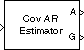# Covariance AR Estimator

Compute estimate of autoregressive (AR) model parameters using covariance method

## Library

Estimation / Parametric Estimation

`dspparest3`

•## Description

The Covariance AR Estimator block uses the covariance method to fit an autoregressive (AR) model to the input data. This method minimizes the forward prediction error in the least squares sense.

The input must be a column vector or an unoriented vector, which is assumed to be the output of an AR system driven by white noise. This input represents a frame of consecutive time samples from a single-channel signal. The block computes the normalized estimate of the AR system parameters, A(z), independently for each successive input frame.

`$H\left(z\right)=\frac{G}{A\left(z\right)}=\frac{G}{1+a\left(2\right){z}^{-1}+\dots +a\left(p+1\right){z}^{-p}}$`

The order, p, of the all-pole model is specified by the Estimation order parameter. To guarantee a valid output, you must set the Estimation order parameter to be less than or equal to half the input vector length.

The top output, `A`, is a column vector of length p+1 with the same frame status as the input, and contains the normalized estimate of the AR model coefficients in descending powers of z.

```[1 a(2) ... a(p+1)] ```

The scalar gain, G, is provided at the bottom output (`G`).

See the Burg AR Estimator block reference page for a comparison of the Burg AR Estimator, Covariance AR Estimator, Modified Covariance AR Estimator, and Yule-Walker AR Estimator blocks.

## Parameters

Estimation order

The order of the AR model, p. To guarantee a nonsingular output, you must set p to be less than or equal to half the input length. Otherwise, the output might be singular.

## References

Kay, S. M. Modern Spectral Estimation: Theory and Application. Englewood Cliffs, NJ: Prentice-Hall, 1988.

Marple, S. L., Jr., Digital Spectral Analysis with Applications. Englewood Cliffs, NJ: Prentice-Hall, 1987.

## Supported Data Types

PortSupported Data Types

Input

• Double-precision floating point

• Single-precision floating point

A

• Double-precision floating point

• Single-precision floating point

G

• Double-precision floating point

• Single-precision floating point

 Burg AR Estimator DSP System Toolbox Covariance Method DSP System Toolbox Modified Covariance AR Estimator DSP System Toolbox Yule-Walker AR Estimator DSP System Toolbox `arcov` Signal Processing Toolbox

## Extended Capabilities

Introduced before R2006a

## SupportGet trial now